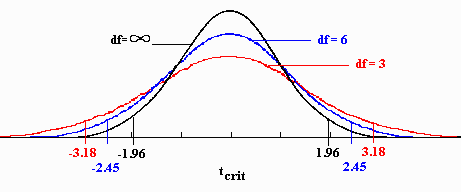## t-Distributions

Suppose we wish to test the claim that a mean of a population is some particular value. Not surprisingly, we look to see if a given sample mean is unusually far removed from this mean. That in turn requires that we look at the distribution of sample means.

With the above as context, suppose $x$ is normally distributed with mean $\mu$ and standard deviation $\sigma$ and we look at the distribution of sample means. We know by the Central Limit Theorem that this distribution of sample means is also normally distributed, but with mean and standard deviation given by $$\mu_{\overline{x}} = \mu \quad \textrm{ and } \quad \sigma_{\overline{x}} = \frac{\sigma}{\sqrt{n}}$$

Consequently, the distribution of test statistics of the form $$z = \frac{\overline{x} - \mu}{\displaystyle{\frac{\sigma}{\sqrt{n}}}}$$ will be a standard normal distribution.

Suppose now that instead of looking at test statistics of the previous form, where we divide by the standard deviation, we look at test statistics of the following form, where we divide by the standard error instead (i.e., we substitute the sample standard deviation, $s$, as an approximation for $\sigma$). $$t = \frac{\overline{x} - \mu}{\displaystyle{\frac{s}{\sqrt{n}}}}$$

When $n$ is large enough, $s$ does a pretty good job at approximating $\sigma$ and the resulting distribution looks very much like a standard normal distribution. Indeed, in the limit the distribution is a standard normal distribution.

However, for smaller samples, when $s$ does not do as good of a job approximating $\sigma$, we have a very different story...

Realizing that $s$ may now over-approximate or under-approximate $\sigma$ by a non-negligible amount, we have added variability into our distribution. Indeed, the resulting distribution will have $\sigma > 1$, which disagrees with the standard normal distribution.

Worse than that, even the shape of the distribution changes! With the added variability, there is more area in the tails of the distribution than a standard normal distribution.

The change in the shape of the distribution of these test statistics $t$ is more pronounced when the sample size $n$ is very small, as in these cases $s$ does a very poor job at approximating $\sigma$.

The distribution so formed is known as a $t$-distribution. Similar to $\chi^2$ distributions, $t$-distributions are a family of distributions, each with an associated degrees of freedom. When we use the sample standard deviation, $s$, as an approximation to $\sigma$ and $n$ is the sample size, the related $t$-distribution has $n-1$ degrees of freedom. As the degrees of freedom get smaller, the boundaries of the middle 95% are pushed outwards, away from zero, as the below graphic shows.Note, $t$-distributions are still roughly bell-shaped (just with fatter tails and a lower center), and are symmetric about $0$, just as the standard normal distribution is. Indeed, as the degrees of freedom increases, the $t$-distribution approaches the standard normal distribution. It also does this rather quickly, such that when the degrees of freedom is 30 or greater, the t-distribution and the standard normal distribution are almost identical.